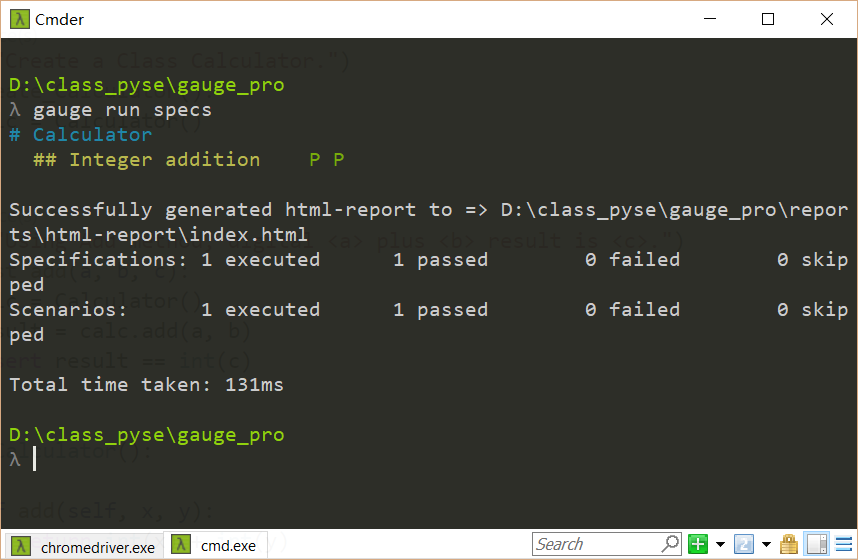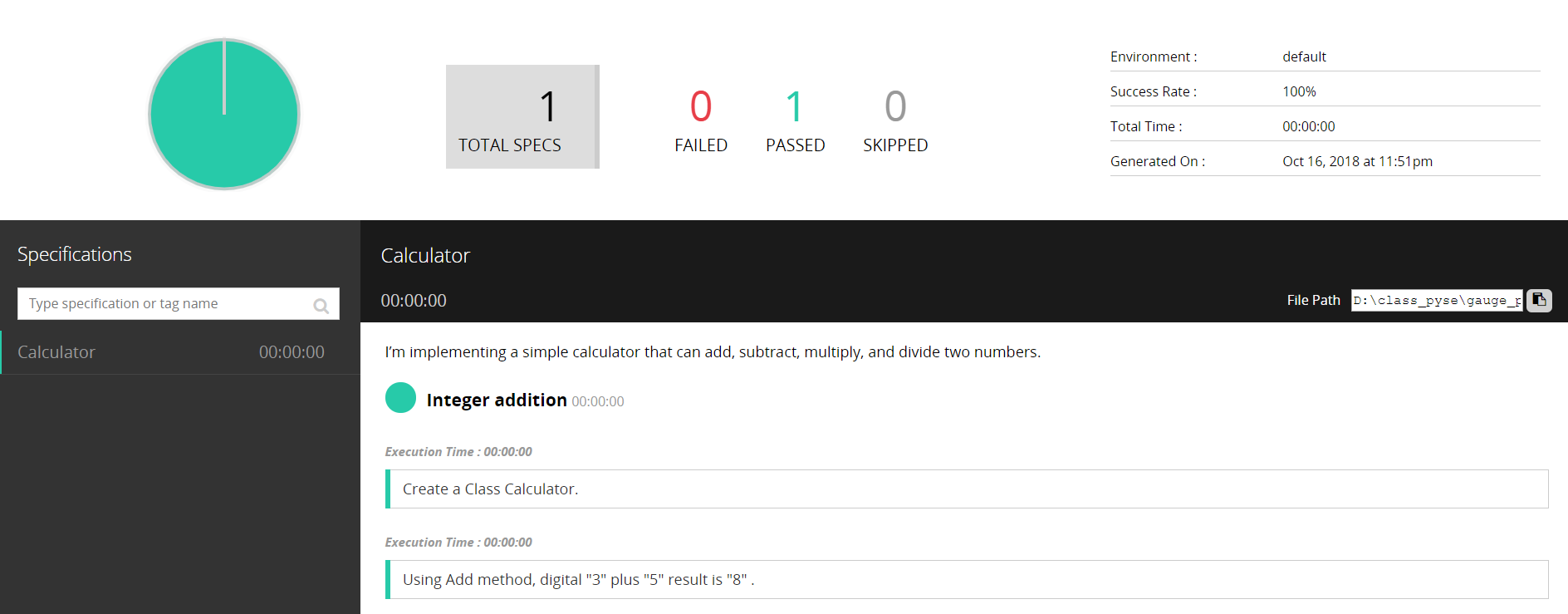## 初识gauge自动化测试框架（二）

2018-10-23 23:31 虫师 阅读(...) 评论(...) 编辑 收藏

Gauge本质上一个BDD（Behavior Driven Development）测试框架。所以，首先你要了解BDD的操作方式。

BDD包含两部分，一部分是： 软件行为描述。另一部分是： 针对描述编写测试代码

# 计算器

## 测试加法

* 创建Calculator类。
* 相使用add方法，计算3 加5 的结果为8。

# Calculator

I'm implementing a simple calculator that can add,
subtract, multiply, and divide two numbers.

* Create a Class Calculator.

* Using Add method, digital "3" plus "5" result is "8".


# -- FILE: features/example.feature
Feature: Showing off behave

Scenario: Run a simple test
Given we have behave installed
When we implement 5 tests
Then behave will test them for us!


http://mahua.jser.me/

from getgauge.python import step

@step("Create a Class Calculator.")
def create_Calculator():
calc = Calculator()

@step("Using Add method, digital <a> plus <b> result is <c>.")
def test_add(a, b, c):
calc = Calculator()
result = calc.add(a, b)
assert result == int(c)

class Calculator():

def add(self, x, y):
return int(x) + int(y)……

## Test addition big number

* Create a Class Calculator.

* Using Add method, digital "301" plus "578" result is "879".


https://getgauge-examples.github.io/